# 【理论+案例实战】Python数据分析之逻辑回归（logistic regression）

sigmoid函数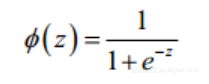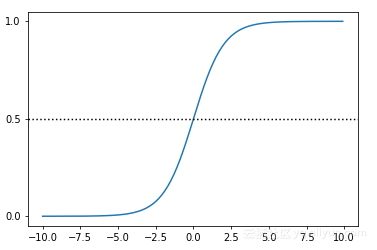（当z为0的时候，函数值为0.5；随着z的增大，函数值逼近于1；随着z的减小，函数值逼近于0.）

import numpy
import math
import matplotlib.pyplot as plt

def sigmoid(x):
a = []
for item in x:
a.append(1.0/(1.0 + math.exp(-item)))
return a

x = numpy.arange(-10, 10, 0.1)
y = sigmoid(x)
plt.plot(x,y)
plt.yticks([0.0, 0.5, 1.0])
plt.axhline(y=0.5, ls='dotted', color='k')
plt.show()


sigmoid函数很适合做我们刚才提到的二分类的分类函数。假设输入数据的特征是(x0, x1, x2, ..., xn)，我们在每个特征上乘以一个回归系数 (w0, w1, w2, ... , wn)，然后累加得到sigmoid函数的输入z：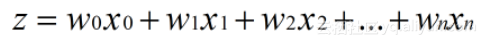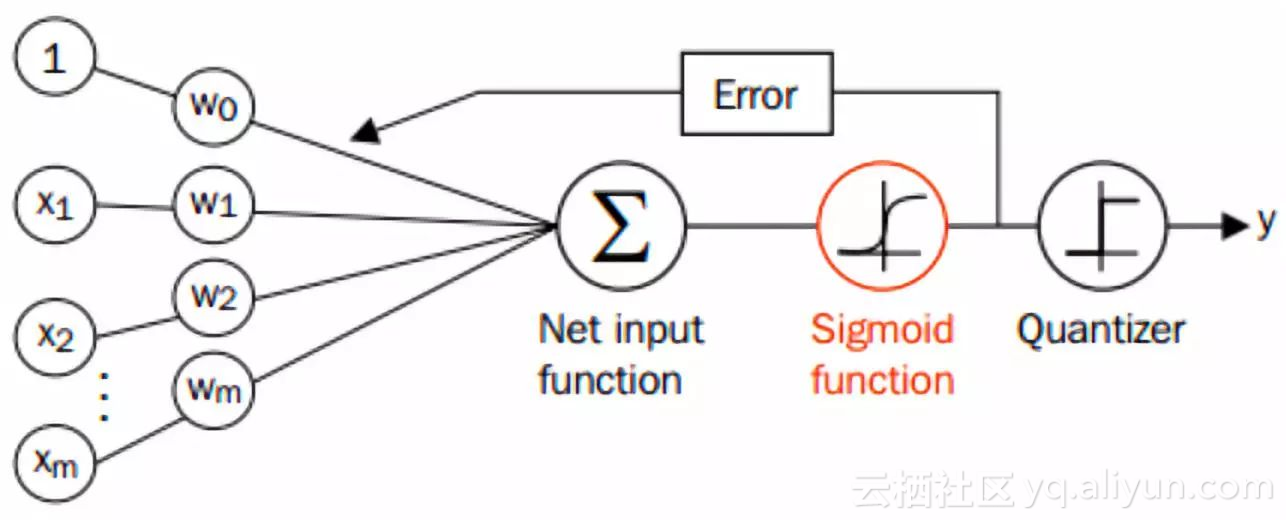http://www.cnblogs.com/kevinGaoblog/archive/2012/03/29/2424346.html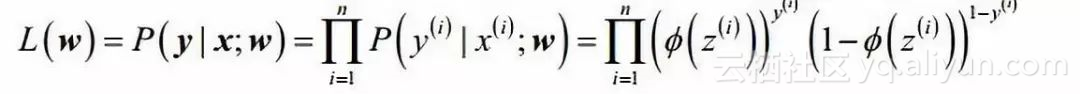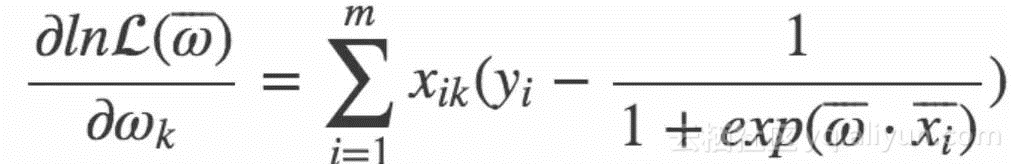import pandas as pd
import statsmodels.api as sm
import pylab as pl
import numpy as np

# 浏览数据集
#0      0  380  3.61     3
#1      1  660  3.67     3
#2      1  800  4.00     1
#3      1  640  3.19     4
#4      0  520  2.93     4

# 重命名'rank'列，因为dataframe中有个方法名也为'rank'
df.columns = ["admit", "gre", "gpa", "prestige"]

#数据统计情况
print (df.describe())
#count  400.000000  400.000000  400.000000  400.00000
#mean     0.317500  587.700000    3.389900    2.48500
#std      0.466087  115.516536    0.380567    0.94446
#min      0.000000  220.000000    2.260000    1.00000
#25%      0.000000  520.000000    3.130000    2.00000
#50%      0.000000  580.000000    3.395000    2.00000
#75%      1.000000  660.000000    3.670000    3.00000
#max      1.000000  800.000000    4.000000    4.00000

#prestige   1   2   3   4
#0         28  97  93  55
#1         33  54  28  12


# 将prestige设为虚拟变量
dummy_ranks = pd.get_dummies(df['prestige'], prefix='prestige')
#   prestige_1  prestige_2  prestige_3  prestige_4
#0           0           0           1           0
#1           0           0           1           0
#2           1           0           0           0
#3           0           0           0           1
#4           0           0           0           1


# 除admit、gre、gpa外，加入了上面常见的虚拟变量（注意，引入的虚拟变量列数应为虚拟变量总列数减1，减去的1列作为基准）
data = df[cols_to_keep].join(dummy_ranks.ix[:, 'prestige_2':])
#  admit  gre   gpa  prestige_2  prestige_3  prestige_4
#0      0  380  3.61           0           1           0
#1      1  660  3.67           0           1           0
#2      1  800  4.00           0           0           0
#3      1  640  3.19           0           0           1
#4      0  520  2.93           0           0           1

# 需要自行添加逻辑回归所需的intercept变量
data['intercept'] = 1.0


# 指定作为训练变量的列，不含目标列admit
train_cols = data[data.columns[1:]]
# sigmoid函数
def sigmoid(inX):  #sigmoid函数
return 1.0/(1+np.exp(-inX))
#梯度上升求最优参数
dataMatrix=np.mat(dataMat) #将读取的数据转换为矩阵
classLabels=np.mat(labelMat).transpose() #将读取的数据转换为矩阵
m,n = np.shape(dataMatrix)
alpha = 0.00001  #设置梯度的阀值，该值越大梯度上升幅度越大
maxCycles = 300 #设置迭代的次数，一般看实际数据进行设定，有些可能200次就够了
weights = np.ones((n,1)) #设置初始的参数，并都赋默认值为1。注意这里权重以矩阵形式表示三个参数。
for k in range(maxCycles):
h = sigmoid(dataMatrix*weights)
error = (classLabels - h)     #求导后差值
weights = weights + alpha * dataMatrix.transpose()* error #迭代更新权重
return weights

#得到权重
#print (weights)


# 在这边为方便，我们将训练集拷贝一份作为预测集（不包括 admin 列）
import copy
test_data = copy.deepcopy(data)

# 预测集也要添加intercept变量
test_data['intercept'] = 1.0

# 数据中的列要跟预测时用到的列一致
predict_cols = test_data[test_data.columns[1:]]

# 进行预测，并将预测评分存入 predict 列中
predict=[]
test=np.mat(predict_cols)
for i in test:
sum=sigmoid(i*np.mat(weights))
print (sum)
if sum <= 0.5:
predict.append('0')
else:
predict.append('1')
test_data['predict']=predict

#计算预测准确率
predict_right=0
for i in range(0,400):
predict_right=1+predict_right
else:
predict_right=predict_right
print ("预测准确率:")
print ("%.5f" %(predict_right/400))
#预测准确率:
#0.68250


def stocGradAscent(dataMatrix,classLabels):
m,n=shape(dataMatrix)
alpha=0.01
weights=ones(n)
for i in range(m):
h=sigmoid(sum(dataMatrix[i] * weights))#数值计算
error = classLabels[i]-h
weights=weights + alpha * error * dataMatrix[i] #array 和list矩阵乘法不一样
return weights


def stocGradAscent1(dataMatrix,classLabels,numIter=150):
m,n=shape(dataMatrix)
weights=ones(n)
for j in range(numIter):
dataIndex=list(range(m))
for i in range(m):
alpha=4/(1+i+j)+0.01#保证多次迭代后新数据仍然具有一定影响力
randIndex=int(random.uniform(0,len(dataIndex)))#减少周期波动
h=sigmoid(sum(dataMatrix[randIndex] * weights))
error=classLabels[randIndex]-h
weights=weights + alpha*dataMatrix[randIndex]*error
del(dataIndex[randIndex])
return weights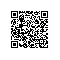Python爱好者使用钉钉扫一扫加入圈子
+ 订阅